Courses

# Previous Year Questions: Centre of Mass

## 11 Questions MCQ Test Physics Class 11 | Previous Year Questions: Centre of Mass

Description
This mock test of Previous Year Questions: Centre of Mass for JEE helps you for every JEE entrance exam. This contains 11 Multiple Choice Questions for JEE Previous Year Questions: Centre of Mass (mcq) to study with solutions a complete question bank. The solved questions answers in this Previous Year Questions: Centre of Mass quiz give you a good mix of easy questions and tough questions. JEE students definitely take this Previous Year Questions: Centre of Mass exercise for a better result in the exam. You can find other Previous Year Questions: Centre of Mass extra questions, long questions & short questions for JEE on EduRev as well by searching above.
QUESTION: 1

### Two block of masses 10 kg and 4 kg are connected by a spring of negligible mass and placed on a frictionless horizontal surface. An impulse gives a velocity of 14 m/s to the heavier block in the direction of the lighter block. The velocity of the centre of mass is    [IIT(Scr.)-2002]

Solution:

Velocity of heavier block after the impulse = 14m/s
Velocity of lighter block after the impulse = 0 m/s
Thus velocity of COM = 14 x 10 + 0 x 4  / 10 + 4 = 10m/s

QUESTION: 2

Solution:
QUESTION: 3

### The balls, having linear momenta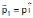and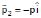, undergo a collision in free space. There is no external force acting on the balls. Let and be their final momenta. The following options(s) is (are) NOT allowed for any non-zero value of p, a1, a2, b1, b2, c1 and c2    [JEE 2008]

Solution:

As given
P=P Î
P2​​=−PÎ
∴  Net Linear Momentum first before collision
Pi=P1​​+P2​​=PÎ−PÎ=0
∵  No external force is acting on the balls, momentum will remain conserved.
Pf′​=P1​​+P2​​=0
∴ In option (A)=P1​​+P2​​=(a1​+a2​)Î+(b1​+b2​)Î+c1​k=/0
Qc1​ in non-zero
∴  P1​​+P2​​=(a1​+a2​)Î +2b1​Î =0
Q b1​ is non zero

QUESTION: 4

A small block of mass M moves on a frictionless surface of an inclined plane, as shown in figure. The angle of the incline suddenly changes from 60° to 30° at point B. The block is initially at rest at A. Assume that collisions between the block and the incline are totally inelastic (g = 10 m/s2). Figure :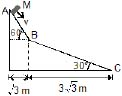The speed of the block at point B immediately after it strikes the second incline is

[JEE 2008]

Solution: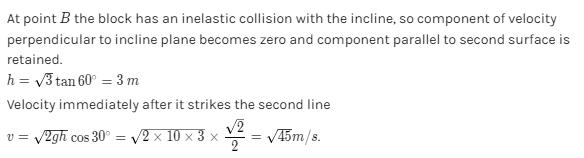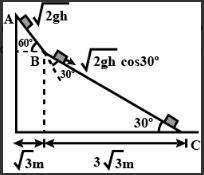QUESTION: 5

A small block of mass M moves on a frictionless surface of an inclined plane, as shown in figure. The angle of the incline suddenly changes from 60° to 30° at point B. The block is initially at rest at A. Assume that collisions between the block and the incline are totally inelastic (g = 10 m/s2). Figure :The speed of the block at point C, immediately before it leaves the second incline is

[JEE 2008]

Solution:

At point B since the block undergoes inelastic collision, the component of velocity perpendicular to the incline becomes zero and only the component of velocity parallel to the incline is left.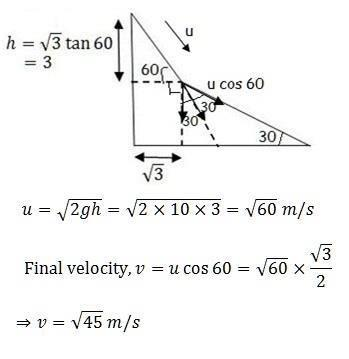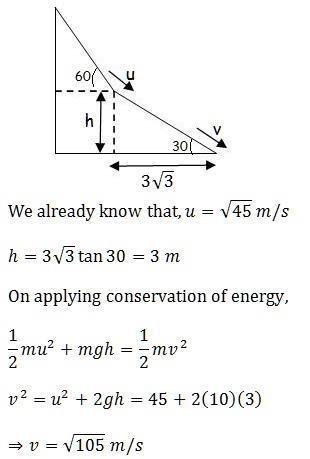QUESTION: 6

A small block of mass M moves on a frictionless surface of an inclined plane, as shown in figure. The angle of the incline suddenly changes from 60° to 30° at point B. The block is initially at rest at A. Assume that collisions between the block and the incline are totally inelastic (g = 10 m/s2). Figure :

[JEE 2008]If collision between the block and the incline is completely elastic, then the vertical (upward) component of the velocity of the block at point B, immediately after it strikes the second incline is

Solution:

v=√2g(3)=√60m/s
Along the plane velocity just after collision
vB=v cos 30°=√45m/s
vy= v sin 30° cos 30° − v cos 30° cos 60°
vy=0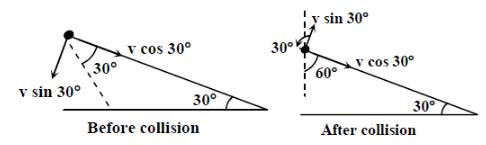QUESTION: 7

Look at the drawing given in the figure which has been drawn with ink of uniform line-thickness. The mass of ink used to draw each of the two inner circles, and each of the two line segments is m. The mass of the ink unsed to draw the outer circle is 6m. The coordinates of the centres of the different parts are outer circle (0, 0), left inner circle (_a, a), right inner circle (a, a), vertical line (0, 0) and horizontal line (0, _ a). The y-coordinate of the centre of mass of the ink in this drawing is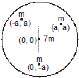[JEE 2009]

Solution: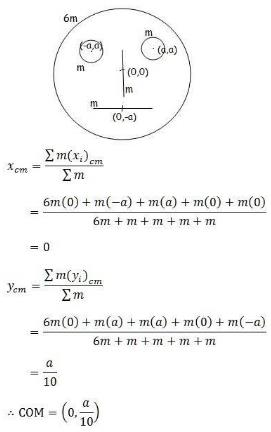QUESTION: 8

Two small particles of equal masses start moving in opposite directions from a point A in a horizontal circle orbit. Their tangential velocities are v and 2v, respectively, as shown in the figure. Between collisions, the particles move with constant speeds. After making how many elastic collisions, other than that at A, these two particulars will again reach the point A ?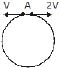[JEE 2009]

Solution:

First collision will occur at an angle of 1200 from the starting point since one particle will cover angle twice of the other one and the sum of the two angles will be 3600. Next, due to elastic collision, velocities will exchange. Similarly, the next collision will occur at an angle 1200 from this position. Then the next collision will occur at A. Hence there will be 2 collisions before meeting again at A.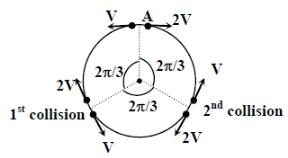QUESTION: 9

Three objects Aand are kept in a straight line on a frictionless horizontal surface. These have masses m, 2and m, respectively. The object moves towardswith a speed 9 ms-1 and makess an elastic collision with it. There after, makes completely inelastic collision with C. All motions occur on the same straight line. Find the final speed (in ms-1 ) of the object C.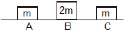[JEE 2009]

Solution:

There is a quantity known as coefficient of restitution which can be defined as the ratio of relative velocity of separation (after collision) and relative velocity of approach (before collision). Mathematically it can be given as shown below. Also for a completely elastic collision, e = 1 and for a completely inelastic collision, e = 0.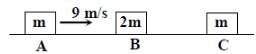After 1st collision
mvA​=mv′​A+2mv′B

−1=v′B​−v′A/0−vA ​​⇒v′B​=6m/s

After the 2nd collision
2mv′​B=(2m+m)VC​​⇒vC​=2​v’B/3​⇒vC​=4m/s

*Multiple options can be correct
QUESTION: 10

A point mass of 1 kg collides elastically with a stationary point mass of 5 kg. After their collision, the 1 kg mass reverse its direction and moves with a speed of 2 ms-1. Which of the following statement(s) is (are ) correct for the system of these two masses ?

[JEE 2010]

Solution:

By using the conservation of linear momentum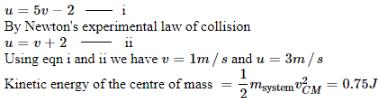Also, the total momentum is 3 kg m/s

*Multiple options can be correct
QUESTION: 11

A ball of mass 0.2 kg rests on a vertical post of height 5 m. A bullet of mass 0.01 kg traveling with a velocity V m/s in a horizontal direction, hits the centre of the ball. After the collision, the ball and bullet travel independently. The ball hits the ground at a distance of 20 m and the bullet at a distance of 100 m from the foot of the post. The initial velocity V of the bullet is

[JEE 2011]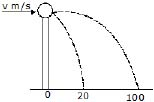Solution: